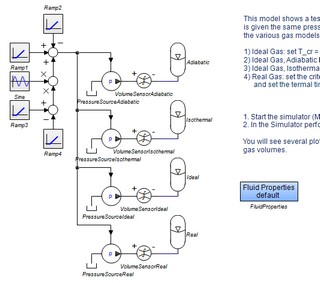﻿ 20-sim webhelp > Language Reference > Keywords > OneUp

# oneup

The keyword Oneup is used to restrict the scope of global parameters and variables. It allows you to use submodels to define the values of global parameters or global variables as an alternative to the Globals Relations Editor.

## Example

In the example model Accumulator Test (type Accumulator Test in the search box to find it in the Examples library) four hydraulic circuits are used. All models in the circuits share the same parameters for the fluid properties such as density, bulk modulus etc. This is done by making them global.The blue submodel Fluid Properties, defines the values of these global parameters. The model internally used the OneUp keyword. The equations of the submodel are:

parameters

real oneup kin_viscosity  (range= <0,>) = 2.7e-5 {m2/s};

real oneup rho (range= <0,>) = 865.0 {kg/m3};

real oneup B (range= <0,>) = 1.6e9 {Pa};

real oneup p_vapour (range= <-1e5,>) = -99900.0 {Pa};

The keyword OneUp limits the scope of a global parameter or variable to the branch that shares contains the submodel where it is defined. In this example this is the model layer that contains the Fluid Properties model and all of the hydraulic circuits that you see.

## OneUp

The keyword oneup should be used after the type (real, boolean, integer) and before the name of the parameter or variable.

## Note

The keywords oneup and global are mutually exclusive. Only one can be used.